# Date and Time

In this chapter, you will practice some date and time functions.

Open a blank workbook for this chapter.

## The TODAY Function

The TODAY function will put the current date in the cell. To use the TODAY function, use the following steps:

1. Select an empty cell.
2. Type =TODAY().
1. This function does not require any arguments, so you can just close the parentheses without anything in them.
3. Press Enter.
1. Excel will enter the current date into the cell.

## The NOW Function

The NOW function will put both the current date and the current time in the cell. To use the NOW function, use the following steps:

1. Select an empty cell.
2. Type =NOW().
1. Like the TODAY function, the NOW function does not require any arguments, and the parentheses can be left empty.
3. Press Enter.
1. Excel will enter the current date and time into the cell.

The time that is entered, however, is not real time. The time only updates whenever the cell recalculates. If you were to select the cell, select the formula bar, and press Enter again, the cell would update to the most current time. Sometimes, if another cell recalculates, this cell will also recalculate. However, the time in this cell is not going to tick through every minute and every second.

## The YEAR Function

Next, we will use the YEAR function, which requires a serial number. A serial number is how Excel interprets dates. If you reference the date from the TODAY function, it will automatically format it as a date. If you use the dropdown menu in the Number section of the Home tab and format the date as General, the date turns into a number. (See Figures 31.1 and 31.2

This number is how Excel interprets dates. They are serial numbers. Each day is an additional number on the serial number. If you made a function in the next cell that added 1 to the cell with the serial number and then formatted the result as a date, Excel would input tomorrow’s date into the cell. You will notice that the time in the Now data updates when the cell containing tomorrow’s date had recalculated.

To use the YEAR function, use the following steps:

1. Select an empty cell.
2. Type =YEAR(.
3. Select the cell that contains the date from the TODAY function.
4. Close the parentheses.
1. For this exercise, the whole formula should read =YEAR(C2)
5. Press Enter.
1. Excel will return with the year of today’s date.
1. For example, the YEAR function in the figures for this chapter would return with 2021.

Keep in mind that this function returns the year as a number, not a date. It’s not returning a date with only the year showing. To make a date only show the year, use the following steps:

1. Select an empty cell, and reference the date we got from the TODAY function.
2. To open the cell’s Format menu, right-click and choose Format or use Control + 1 (PC) or Command + 1 (Mac).
3. Under Category, choose Custom. (See Figure 31.3)
4. In the Type box, type YYYY. (See Figure 31.3)
1. This will show only the year of the date.
5. Select OK.
1. Excel will keep it formatted as a date, but it will only show the year.

However, this is not the same as what showed up when we used the YEAR function the first time. In this example, the function returned with the number, 2,021, not a date. If you were to type = and test to see if the two results of 2021 were the same, Excel would return with FALSE. (See Figure 31.4)

## The MONTH Function

The MONTH function will do something similar. To use the MONTH function, use the following steps:

1. Select the cell next to Month, and type =MONTH(.
2. Select the date that was returned from the TODAY function. (See Figure 31.5)
3. Make sure to close the parentheses.
4. Press Enter
1. Excel will return with the number of the month.

## The DAY Function

The DAY function works the same way as well. To use the DAY function, use the following steps:

1. Select the cell next to Day, and type =DAY(.
2. Select the date that was returned from the TODAY function.
3. Make sure to close the parentheses.
4. Press Enter.
1. Excel will return with the number of the day.
1. We give Excel a serial number, and it returns with a number.
2. In the example from the figures, this would be 6.

## The HOUR Function

The HOUR function works in a similar way, but there is something different. To use the HOUR function, use the following steps:

1. Select the cell next to Hour, and type =HOUR(.
2. Select the date that was returned from the TODAY function.
3. Make sure to close the parentheses.
4. Press Enter.

You will notice that Excel returned with 0. If you press Control + 1 (PC) or Command + 1 (Mac) and select Custom, you will see that this is because the Type box does not include the hour and the minute. To fix this, add hh:mm a/p and select OK (See Figure 31.6). This will add the time in a.m. or p.m. in the TODAY function. Because the TODAY function doesn’t have a time attached to it, it will just show 12:00 a.

To get the result we want, we need to use the HOUR function on the data returned from the Now function. To do this, use the following steps:

1. Select the cell next to Hour, and type =HOUR(.
2. Select the date that was returned from the Now function.
3. Make sure to close the parentheses.
4. Press Enter.
1. Excel will return with the hour in the Now result.
1. In the example from the figures, this would be 20.

## The DATE Function

Next is the DATE function, and this one works in reverse. You need to tell it a year as a number, a month as a number, and a day as a number. To use the DATE function, use the following steps:

1. Select the cell next to Date, and type =DATE(.
2. Select the data that was returned from the YEAR function.
3. Add a comma, and select the data returned from the MONTH function.
4. Add a comma, and select the data returned from the DAY function.
5. Make sure to close the parentheses. (See Figure 31.7)
6. Press Enter.
1. Excel will return with a date formatted just like what the TODAY function returned.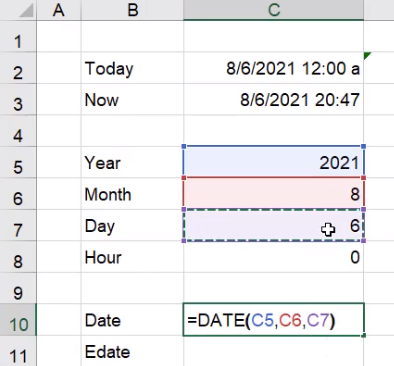Figure 31.7

## The EDATE Function

In addition to having a specific date, the EDATE formula allows you to take a certain starting date and then add or subtract a certain number of months from it. To add months using the EDATE function, use the following steps:

1. Select the cell next to Edate, and type =EDATE(.
2. Select the date from the Date formula.
3. Add a comma, and type 1.
1. This tells Excel that you want to add one month to the original date.
4. Make sure to close the parentheses. (See Figure 31.8)
5. Press Enter
1. Excel will return with the serial number for the new date.
2. Use the format painter to apply the Date formatting to the EDATE result.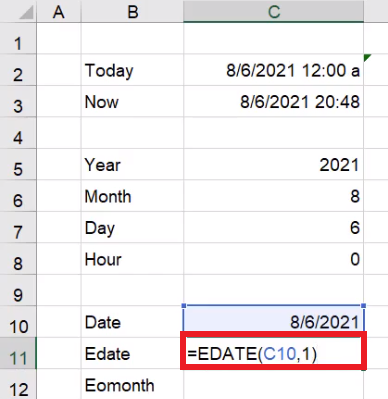Figure 31.8

You could try to get the same result by writing a formula that adds 30 to the original date, but since each month has a different number of days, it will not always be accurate. Using the EDATE function will be more accurate.

To subtract months using the EDATE function, use the following steps:

1. Select the next empty cell next to Edate, and type =EDATE(.
2. Select the date from the Date formula.
1. This is the start date.
3. Add a comma, and type -3.
1. A negative number tells Excel you want to subtract three months from the original date.
4. Make sure to close the parentheses. (See Figure 31.9)
5. Press Enter.
1. Excel will return with the serial number for the new date.
2. Use the format painter to apply the Date formatting to the EDATE result.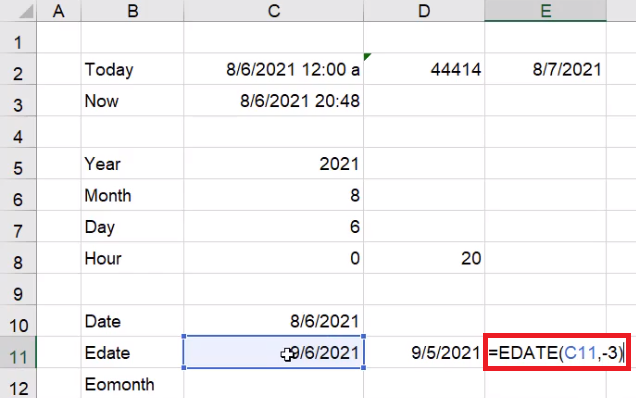Figure 31.9

## The EOMONTH Function

The EOMONTH formula stands for “end of month.” Given a certain start date, the EOMONTH formula adds a certain amount of months. To use the EOMONTH function, use the following steps:

1. Select the cell next to EOMONTH, and type =EOMONTH(.
2. Select the date from the EDATE formula.
1. This is the start date.
3. Add a comma, and type 0.
1. This tells Excel that you want the last day of the month from the start date.
4. Make sure to close the parentheses. (See Figure 31.10)
5. Press Enter.
1. Excel will return with the serial number for the new date.
1. Use the format painter to apply the Date formatting to the EOMONTH result.
2. Once it is formatted as a date, Excel will return with the last day of the start date.
1. In the example from the figures, this would be 9/30/2021.

Instead of going to the end of the current month, you can also go to the end of the next month. To do this, use the following steps:

1. Select the next empty cell next to EOMONTH, and type =EOMONTH(.
2. Select the date from the EDATE formula.
1. This is the start date.
3. Add a comma, and type 1.
1. This tells Excel you want the last day of the month one month from the current date.
4. Make sure to close the parentheses. (See Figure 31.11)
5. Press Enter
1. Excel will return with the serial number for the new date.
1. Use the format painter to apply the Date formatting to the EOMONTH result.
2. Once it is formatted as a date, Excel will return with the last day of the start date.
1. In the example from the figures, this would be 10/31/2021.

Always make sure that the formatting is correct. Excel thinks of dates as numbers, but we want to see them formatted as a date. Here is another example of date formatting:

1. Select a cell that contains a date.
2. Press Control + 1 (PC) or Command + 1 (Mac) to open the Format Cells dialog box.
3. Under Category, select Custom.
1. This will allow you to customize the formatting of your dates.
2. There is also a Date category, which will give you a list of pre-built options, but we will not use it for this example.
4. In the Type box, type yyyy.
1. This tells Excel you want the date first, with all four digits.
5.  Add a space, and type mmmm
1. This tells Excel you want the full name of the month.
1. Typing mmm will give you an abbreviation of the month.
6. Add a space, and type dd. (See Figure 31.12)
1. This tells Excel you want the day to always show up as double digits.
1. Single-digit days will have a zero in front. For example, the first day would appear as 01.
7. Select OK.
1. Excel will change the formatting to the custom format.
2. For example, in the example from the figures, the date would now read as follows: 2021 September 30.

There are a lot of ways you can customize how these dates are displayed, but what’s important to remember is the underlying value. In this case, it’s a date, so it’s going to appear as a serial number as seen in Figure 31.2. In the case of the YEAR function, it appears as a number, so you can’t actually format it with any of the date formats. If you tried to format 2021 as a date, Excel would read it as the serial number for the year 1905, which is not what you want.

## The WEEKDAY Function

Next, we’ll look at the WEEKDAY function. This gives you the number of the day of the week of a certain date. To use the WEEKDAY function, use the following steps:

1. Select the cell next to Weekday, and type =WEEKDAY(.
2. Select the result from the TODAY function.
3. Add a comma to get to the return type.
1. This allows you to choose how Excel counts the days of the week. (See Figure 31.13)
1. The default has Sunday as the first day of the week and Saturday as the last day of the week.
4. Type 1 to set it to the default setting.
5. Make sure to close the parentheses. (See Figure 31.14)
6. Press Enter.
1. Excel will return with the day number of the day of the week.
2. In the example from the figures, Excel would return with 6, meaning the weekday is a Friday.

If you did the same formula and chose a return type where the first day of the week is Thursday, then the formula would return with 2. When we use this formula, we have to be careful which type that we’re referencing. If you just choose the date and don’t enter that second argument, Excel will go with the default of the first return-type option.

## The WEEKNUM Function

The WEEKNUM function tells you the week number of the date in the year. To use this function, use the following steps:

1. Select the cell next to WEEKNUM and type =WEEKNUM(.
2. Select the result from the TODAY function.
3. Add a comma, and type 1 to let the week begin on Sunday.
4. Make sure to close the parentheses. (See Figure 31.15)
5. Press Enter.
1. Excel will return with the week number the TODAY date falls in.
2. In the example from the figures, Excel will return with 32, meaning the inputted date falls in the 32nd week of the year.

## The WORKDAY Function

The next function that we’ll look at is the WORKDAY function. You’ll notice there are actually two options: WORKDAY and WORKDAY.INTL. (See Figure 31.16)

Some prefer to use the WORKDAY.INTL formula because it’s more extensive in the options that it presents to you, so this is the one we will use in this practice. This function will tell you what date it will be after a number of inputted workdays. To use this formula, use the following steps:

1. Select the cell next to Workday and type WORKDAY.
2. Select the WORKDAY.INTL option. (See Figure 31.16)
3. Select the result from the TODAY function.
1. This is the start date.
4. Add a comma, and type 10.
1. This signifies how many workdays you want to go into the future.
5. Add a comma, and type 1.
1. This is an optional argument, and 1 signifies the default weekend of Saturday and Sunday.
6. Make sure to close the parentheses. (See Figure 31.17)
1. The last parameter has to do with holidays. This could include holidays like Christmas, Easter, or New Year’s Day. However, you have to have that list in a separate set of cells, and the dates have to be the correct year on that list of holidays.
7. Press Enter
1. Excel will return with the serial number for the date.
2. Use the format painter to format it as a date.
3. In the example from the figures, Excel will return with 8/20/2021.

You may be wondering why it took all the way from the 6th to the 20th to get through 10 workdays, because that is longer than 10 days. This is because the WORKDAY function excludes Saturdays and Sundays when it counts out the ten days. (See Figure 31.18)

## Applying Our New Knowledge

So that’s a quick introduction to a number of different date and time functions. Let’s go through some examples of how we can use these in projects or potential work scenarios.

Using the TODAY Function

The first example uses the TODAY function. Let's say that you have a report that has a number of days in it. Let’s just say for this month, we start on the first of the month. Use the following steps:

1. Select an empty cell, and type 8/1.
2. Press Enter.
1. Excel will enter 1-Aug into the cell.
3. In the next cell down, type =.
4. Select 1-Aug.
6. Press Enter.
7. Click and drag the little box in the bottom-right corner of the cell to make a list that goes down to 15-Aug.

Highlighting Numbers for a Specific Date

Next, let’s try highlighting a specific date. For this example, we’ll use a sales report and forecast using a random number between 100 and 1000.

1. Enter the word Sales in cell IA.
2. In cell I2, next to the first date, type =RANDBETWEEN(100,1000).
3. Select the corner of the formulated cell and drag it down to be even with the last date in the column to the left.

We’d like to specifically highlight the day that we’re currently on (August 6) so we can get the actual number of sales; all days listed after our current date will be considered forecasted numbers. It’ll be easier to differentiate from the actual number of sales and forecasted number of sales if we highlight the number for the current date.

1. Select the range I2 through I16.
2. Select Conditional Formatting.
3. Select New Rule.
4. Select the option Use a Formula to Determine Which Cells to Format.
1. In the Edit the Rule Description text box, type =$H2=today()-1. (See Figure 31.19) 5. Select Format and choose a fill color. 6. Select Okay.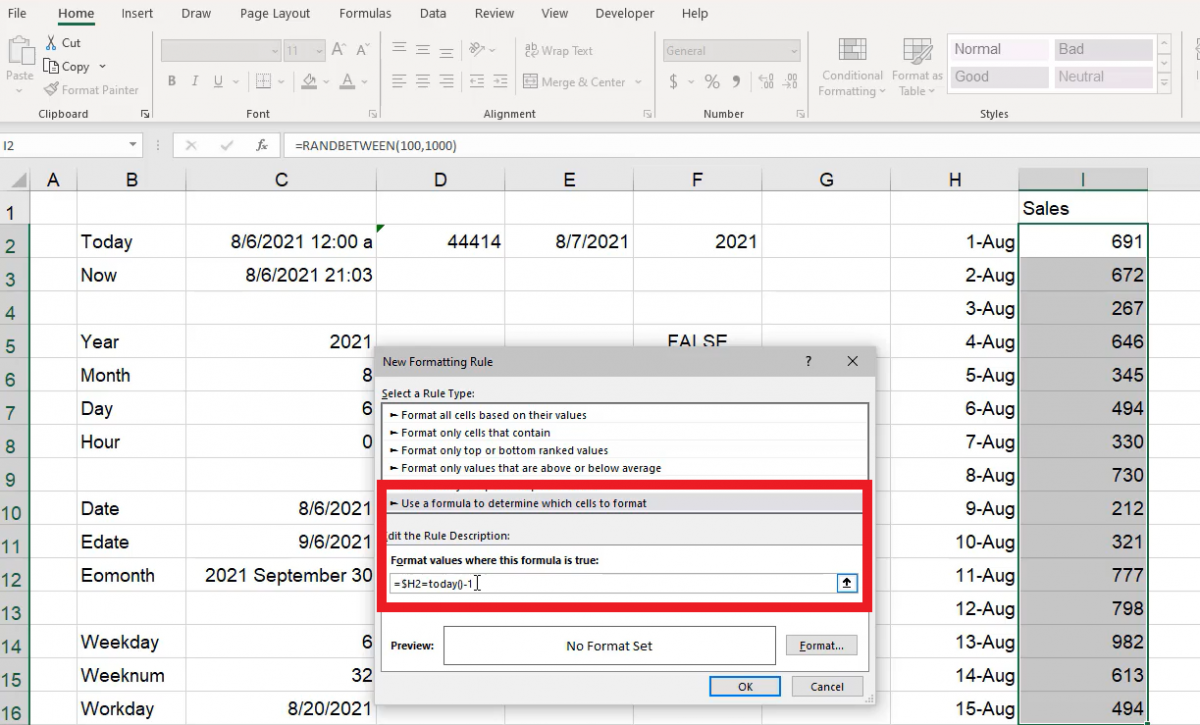Figure 31.19 You should see that yesterday (August 5), would be the last day there are actual numbers for. This is just one example of how you can use the TODAY formula. (See Figure 31.20)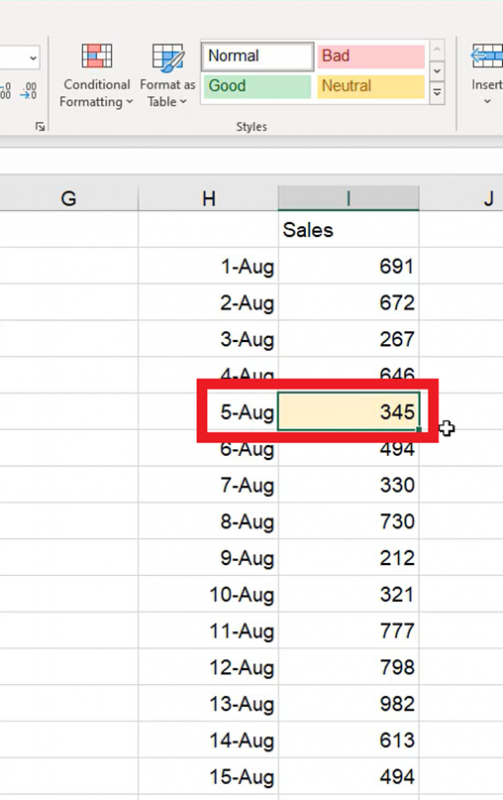Figure 31.20 Setting a Repeated Schedule Let’s look at options on how to set up schedules using the TODAY formula. For this example, we’ll assume that some sort of analysis needs to be completed on a certain day of every month. Let’s say it’s the 15th. We’ll start by using the date function. 1. Select cell K2 and enter =DATE(YEAR(today()),MONTH(today()),15. 2. Select cell K3 and enter =EDATE(K2,1). 3. Select the corner of cell K3 drag it down to K16. You’ll notice that the day shows the 15th of each of the months into the future. If you wanted to know what day of the week it will be, change the format to include the spelled out day of the week. 1. Select the range of cells K2 through K16. 2. Open Format Cells. 3. Select Custom. 4. In the Type text box, type dddd and a space before the month. (See Figure 31.21) 5. Select Okay.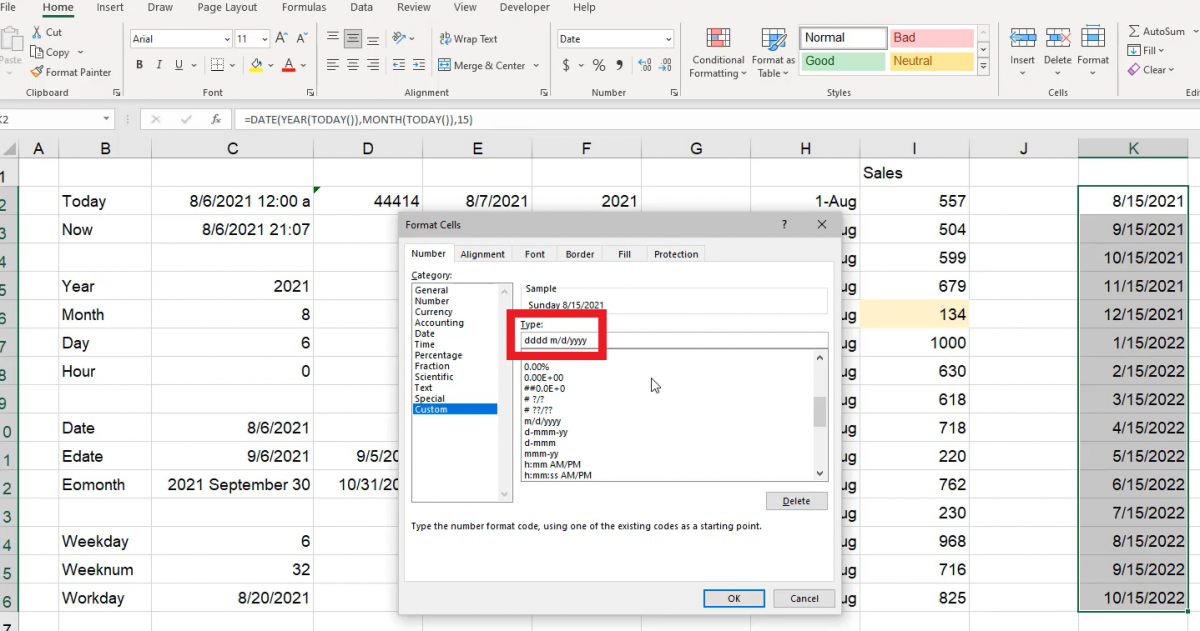Figure 31.21 You may need to extend the K column to see all of the information in the row. Now you should see the day of the week the 15th will land on in the following months. Using EOMONTH The EOMONTH function is helpful if you have a dashboard you need to reference. Let’s say you have a starting day of August 1 and you want to know what day is the end of the month; instead of figuring out how many days each month has, you can have a formula figure it out for you: 1. Select cell F19. 2. Type 8/1 3. Select cell G19. 4. Type =EOMONTH(F19,0) 1. The cell F19 indicates the first of the month and the 0 indicates the end of the month. 5. Select F19, then select the Format Paint tool. 6. With the Format Paint tool still selected, select G19 to correctly format the date.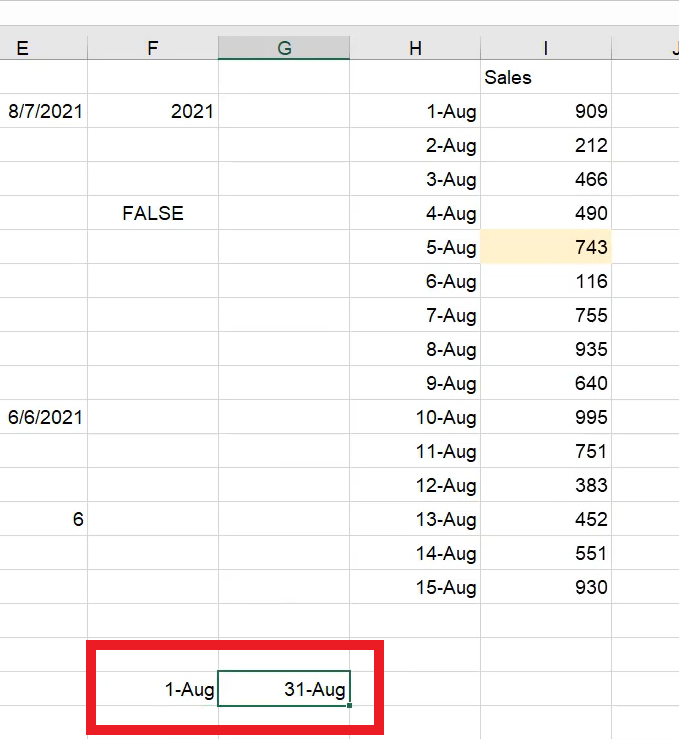Figure 31.22 You should now be able to see the first and last dates of the month (see Figure 31.22); you can put the next month into the first of the month cell and it will automatically update the last date of the month accordingly. This is helpful in SUMIF or COUNTIF formulas if you ever need to sum up a range of sales information, quantity, or orders received throughout a course of given dates. Using WORKDAY.INTL with Holidays A particularly helpful function is the WORKDAY.INTL function. If you’re in project management, this function helps you know what day a project will be completed. It can calculate the workdays and factor in holidays. Complete the following to formulate how long it will take to complete projects within 10 days, 20 days, 50 days, 100 days, and 200 days when factoring in weekends and holidays. First, let’s anchor in the holidays. 1. Indicate the project dates. 1. In cell C22, type 10. 2. In cell C23, type 20. 3. In cell C24, type 50. 4. In cell C25, type 100. 5. In cell C26, type 200. 2. In cell D22, next to the number 10, enter the following formula: 1. =WORKDAY.INTL(today(),C22,1,$C$19:$C\$20). Hit Enter.
2. The anchored cells will be where we will put information about the holidays.
3. Select cell B19 and type New Years. Enter the following formula into C19:
1. =DATE(YEAR(today())+1,1,1). Hit Enter.
4. Select [##] cell and type in Christmas. Enter the following formula into C20:
1. =DATE(YEAR(today()),12,25). Hit Enter.
5. Select C16, and then select Format Painter.
6. Select D22 to format paint the cell to show as a date.
7. Select the corner of cell D22 and drag it down to cell D26.